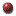10.213
Chemical Engineering Thermodynamics
 Spring 2002 MWF 10, 4-231HomeClass InformationHandoutsProblem SetsExamsExtra ProblemsUseful LinksFeedback last update 05/23/02

Additional Examples

These are older problems; most of them have solutions. Disclaimer: The solutions were made by others before us (current instructors). While the solution methods are generally sound, we are not guaranteeing that they are completely relevant, accurate, and optimal. Do NOT use these problems as reference. Use them as what they are: practice problems.

A few problems are not suitable for us because different semesters may cover some different materials; e.g. colligative property and availability/exergy were not discussed in Spring 2002. Please contact us if you have questions.

 Fall 2000 Spring 2001 1999 PS1,   solution - Kinetic and Potential Energy, Work, Heat, Isothermal Compression PS2,   solution - P-V diagrams, Isobaric Expansion, 1st Law for Closed Systems, Enthalpy Change for constant P Processes PS3,   solution - P-T diagrams, Saturation (vapor pressure), Antoine's Equation PS4,   solution - Non-Ideal Gas Behavior, Virial EOS, Compressibility PS5,   solution - Non-Ideal Gas Behavior, Lee/Kessler Generalized Correlations, Cubic EOS PS6,   solution - Heat Capacity and Temperature, Heat Interactions, Average Heat Capacity for Enthalpy PS7,   solution - Non-Ideal Gass Behavior, Residual Properties, Lee/Kessler Generalized Correlations, lnP vs. H Diagrams PS8,   solution - lnP vs. H diagram, Tables of Thermodynamics Data, Saturation (Vapor Pressure), Theory of Corresponding States, Steam Tables PS9,   solution - Material Balances PS10, solution - Material Balances PS11, solution - Material and Energy Balances PS12, solution - Refrigeration Cycle PS13, solution - Energy Balance PS14, solution - Carnot Cycle, Reversible Cycles PS15, solution - Material and Energy Balance (not Steady State) PS16, solution - Availability / Lost Work PS17, solution - Compressor/Turbine, Steam Table, Lost Work PS18, solution - Partial Molar Property, V-x diagram PS19, solution - Excess Property PS20, solution PS21, solution - Pxy diagram PS22, solution - Flash calculation, Material Balance + Phase Equilibrium PS23, solution - Solution Model from Pxy Data PS24, solution - Bubble Point, Dew Point, Azeotrope PS25, solution - Colligative Property, Freezing Point Depression PS26, solution PS27, solution 1995 PS1,   solution - Heat Capacity, Vapor-Liquid Equilibrium, Cubic EOS, Expansion Work of a Non-Ideal Gas PS2,   solution - Residual Properties, Generalized Correlations, Reduced Properties, Thermodynamic Properties and Partial Derivatives PS3,   solution - Mass Balance (steady state, no reaction) PS4,   solution - Mass Balance (with reaction), Energy Balance PS5,   solution - Energy Balance PS6,   solution - 2nd Law, Coefficient of Performance, Availability, Maximum Work PS7,   solution - Chemical Potential, Isothermal Compression, Antoine's Equation, Raoult's Law PS8,   solution - Mass and Energy Balance, Lewis/Randall Rule, Raoult's Law, Poynting Correction PS9,   solution - Partial Molar Properties, Gibbs-Duhem, Antoine's Equation, Activity Coefficient, Modified Raoult's PS10, solution - Reaction Equilibrium, Independant Reactions, Energy Balance 1994 PS1,   solution - Equations of State, P-V Diagrams, Compression Work, Vapor-Liquid Equilibrium PS2,   solution - Heat of Vaporization, Non-Ideal Gas, Steam Tables, Generalized Correlations PS3,   solution - Mass Balances PS4,   solution - Energy Balances, Binary VLE, Bubble and Dew Point Calculations PS5,   solution - Energy Balances, lnP vs. H Diagrams PS6,   solution PS7,   solution - Binary VLE, T-x and T-y Diagrams, Raoult's Law, Bubble and Dew Point Calculations PS8,   solution - Fugacity, Excess Gibbs Free Energy, Generalized Correlations, Binary VLE PS9,   solution - Models for Excess Gibbs Free Energy, Azeotropes, Antoine's Equation, Henry's Law, Vapor Pressure, P-x and P-y Diagrams Old Exams 1999 Exam 1,   solution - Non-ideal EOS, Work Calculation, Extensive and Intensive Volume, PV Diagram 1999 Exam 3,   solution - Material & Energy Balance, Ideal Work, Excess Property, Phase Equilibrium 1999 Exam 4,   solution - Phase Equilibrium 1995 Exam 1,   solution - Thermodynamic tables and diagrams, Saturated vapor and liquid, Cp(T), S and H calculations, Ideal and Non-ideal gases, Expansion, Virial EOS, PV diagrams 1995 Exam 2,   solution - Turbine expansion, Non-ideal gases, Steam tables, Actual and maximum work, Open system material and energy balances 1995 Exam 3,   solution - Binary VLE, Vapor pressure, Saturdated vapor and liquid, Solid-vapor equilibrium, Fugacity, Virial EOS, Activity coefficient, Heat of mixing 1994 Exam 1,   solution - Cp(T), Virial EOS, H and S calculations, Saturation and Vaporization, Mollier Diagram, Expansions, Non-ideal gas behavior 1994 Exam 2,   solution - Energy balances, ln(P)-H diagrams, Minimum Work 1994 Exam 3,   solution - Change in entropy for mixing, Lost Work, Vapor Pressure, Binary VLE, Dew and bubble points